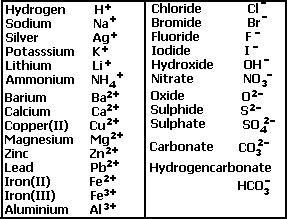Ionic equations

for acid/base reactions

Ionic equations show only the species taking part in the reaction and not the spectator ions.

When writing acid/base ionic equations, remember to fully dissociate the aqueous substance and fully ionise the acids. We will shortly see how this done, but first look at how some acids fully ionise.

nitric acid (HNO3) = H+(aq) + NO3 -(aq)

sulfuric acid (H2SO4) = 2H+(aq) + SO4 -2(aq)

hydrochloric acid (HCl) = H+(aq) + Cl-(aq)

Example
Write the ionic equation for the reaction between nitric acid and calcium carbonate powder.

Step 1) Write the overall balanced equation.
2HNO3(aq) + CaCO3(s) => Ca(NO3)2(aq) + CO2(g) + H2O(l)

Step 2) Dissociate the aqueous compounds and fully ionise the acid.
2H+(aq) + 2NO3-(aq) + CaCO3(s) => Ca+2(aq) + 2NO3-(aq) + CO2(g) + H2O(l)

Step 3) Remove all species that appear on both sides of the equation in the same state.
In this case 2NO3-(aq) will be removed to give the equation below.
2H+(aq) + CaCO3(s) => Ca+2(aq) + CO2(g) + H2O(l)Valency table of common ions

Write the ionic equation for the following reactions

 Hydrochloric acid and solid potassium carbonate Solution Hydrochloric acid and aqueous potassium carbonate Solution Sulfuric acid and solid sodium carbonate. Nitric acid and magnesium metal Hydrochloric acid and magnesium sulfide Sulfuric acid and magnesium oxide powder. Nitric acid and aqueous calcium hydroxide. Hydrochloric acid and aqueous sodium sulfite. Sulfuric acid and solid calcium carbonate Nitric acid and aqueous sodium carbonate.
Solutions